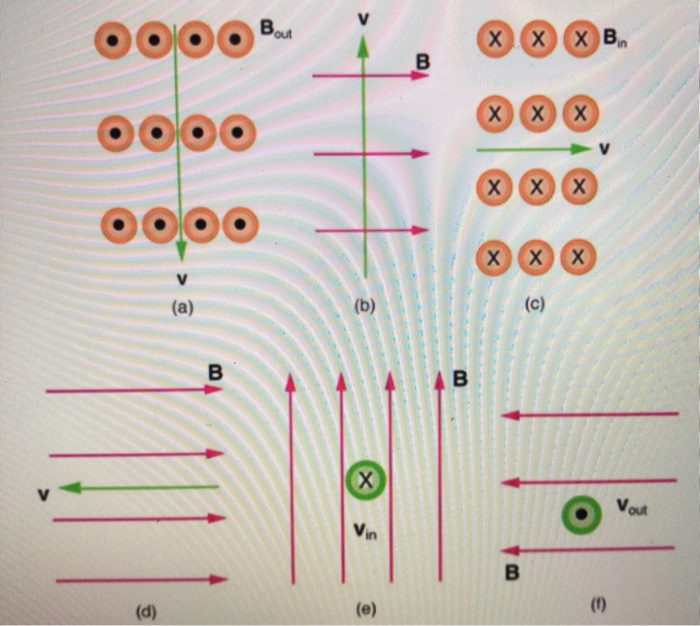# Problem: Consider the magnetic fields and velocities of charged particles shown in the figure.Part (a) What is the direction of the magnetic force on a positive charge that moves as shown in (a)?Part (b) What is the direction of the magnetic force on a positive charge that moves as shown in (b)?Part (c) What is the direction of the magnetic force on a positive charge that moves as shown in (c)?Part (d) What is the direction of the magnetic force on a positive charge that moves as shown in (d)?Part (e) What is the direction of the magnetic force on a positive charge that moves as shown in (e)?Part (f) What is the direction of the magnetic force on a positive charge that moves as shown in (f)?

###### FREE Expert Solution

We'll use the right-hand rule to figure out the direction of the magnetic force.

83% (107 ratings)###### Problem Details

Consider the magnetic fields and velocities of charged particles shown in the figure.Part (a) What is the direction of the magnetic force on a positive charge that moves as shown in (a)?
Part (b) What is the direction of the magnetic force on a positive charge that moves as shown in (b)?
Part (c) What is the direction of the magnetic force on a positive charge that moves as shown in (c)?
Part (d) What is the direction of the magnetic force on a positive charge that moves as shown in (d)?
Part (e) What is the direction of the magnetic force on a positive charge that moves as shown in (e)?

Part (f) What is the direction of the magnetic force on a positive charge that moves as shown in (f)?